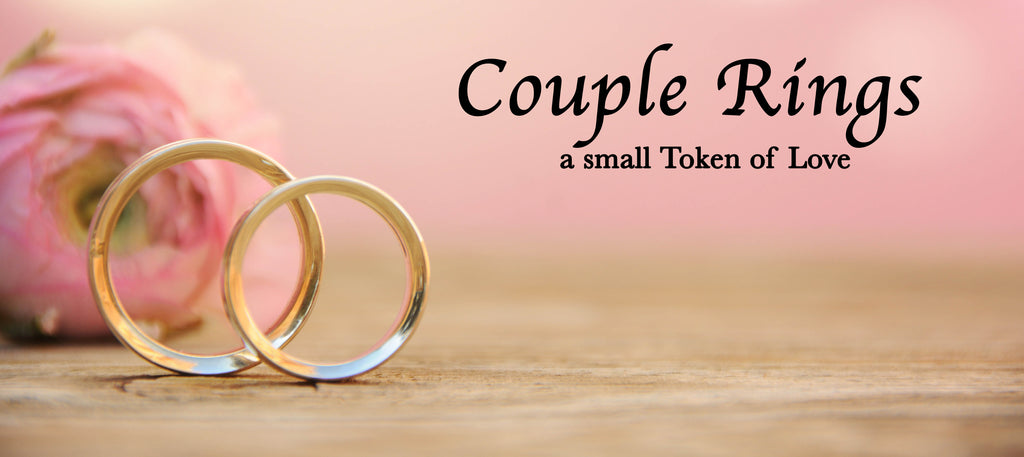To show your partner the affection you share among yourselves.
Filter
Rs. 499.00
Rs. 499.00
Rs. 549.00
Rs. 999.00
Rs. 599.00
Rs. 499.00
Rs. 399.00
Rs. 399.00
Rs. 599.00
Rs. 599.00
Rs. 599.00
Rs. 526.00
Rs. 612.00
Rs. 612.00
Sold Out
Rs. 996.00
Rs. 899.00
Rs. 899.00
Rs. 599.00
Rs. 549.00
Rs. 423.00
Rs. 526.00
Sold Out
Rs. 612.00
Rs. 612.00
Rs. 519.00
Rs. 499.00
Rs. 612.00
Rs. 649.00
Rs. 649.00
Sold Out
Rs. 1,099.00
Rs. 999.00
Rs. 649.00
##### Platinum Plated Elegan...
Rs. 1,996.00 Rs. 599.00
Rs. 499.00
Rs. 599.00
Rs. 599.00
Rs. 499.00
Rs. 599.00
Rs. 599.00